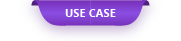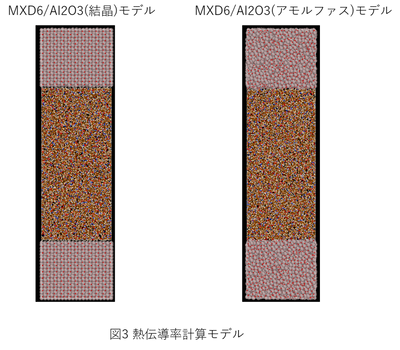In recent years, multi-materialization has been attracting attention for the purpose of reducing...

# Analysis of interfacial thermal resistance at metal-resin junction interface using non-equilibrium molecular dynamics

In recent years, multi-materialization has been attracting attention for the purpose of reducing the weight of structures, and we have been able to understand the microstructure of the interface from the strength analysis of the metal-resin interface using molecular dynamics calculation. It has been shown to be important for increasing strength. In this case, we will introduce an example of analyzing the interfacial thermal resistance at the metal-resin interface using the non-equilibrium molecular dynamics method as an important property other than the bond strength. Exabyte.io has a workflow that allows users to easily create a complicated model of the metal-resin interface, set the force field, and set calculation conditions such as tensile strength analysis and thermal conductivity analysis, so the user can easily set the metal-resin interface. It is possible to perform the simulation of.

### 1. Calculation model and calculation conditions

This time, the resin molecules were PA6 and MXD6, which are thermoplastic resins (Fig. 1), and Al 2 O 3 was examined as the metal surface. We also considered clean and amorphous surfaces as Al 2 O 3 surfaces.

The number of repetitions of the resin molecule was set to n = 10, and the calculation cell was filled so that the specific gravity was 1.13 for PA6 and 1.22 for MXD6. Since the initial structure of the resin molecule is a structure without bending, at the start of the simulation, allow a margin in the length in the surface direction (z direction), fill the molecule, and cell in the z direction so that the desired specific gravity is obtained. The structure was relaxed while deforming (Fig. 2). Figure 3 shows the final computational model used.

LAMMPS was used for all molecular dynamics calculations, OPLS-AA was used for the resin molecule, and PolyPerGen  was used for the force field and atomic charge settings. The Buckinghum potential  was used for Al 2 O 3, and the UFF potential  was used for the interaction between the resin molecule and the Al 2 O 3 surface . In addition, the molecular dynamics calculation conditions for the calculation of thermal conductivity were carried out in the following steps.

(1) Thermal equilibrium of 1ns with NPT ensemble (300K, 1atm) while deforming the cell only in the surface direction (z direction)

(2) 1ns thermal equilibrium with NVT ensemble (300K, 1atm)

③ Perform a non-equilibrium MD calculation of 3ns under a temperature gradient to obtain a constant heat flux.

④ Perform 1ns non-equilibrium MD under temperature gradient and evaluate thermal conductivity.

Figure 4 shows the non-equilibrium MD calculation conditions for thermal conductivity calculation.

### 2. Calculation result

Figure 5 shows the calculation results of the temperature profile of the metal / resin interface. Comparing PA6 and MXD6, it can be seen that MXD6 has a smaller interface temperature gap and better heat transfer at the interface. This means that MXD6 has better adhesion to the metal surface. Comparing the case where the Al 2 O 3 surface is a clean surface (crystal) and the case where it is amorphous, it was confirmed that the temperature gap at the interface is smaller and the adhesion is higher on the amorphous surface.

Figure 6 shows the calculation result of the time change of heat flux. There was no significant difference in the heat flux generated by the temperature gradient between the clean surface and the amorphous surface, but the result was that the heat flux was larger on the amorphous surface, and the amorphous surface transferred heat to the resin. I found it easy to convey.

Table 1 shows the calculation results of the interfacial thermal resistance and the thermal conductivity of the resin evaluated from Fig. 5 and Fig. 6. The thermal conductivity of the resin molecule was close to that of the actual measurement (0.35 to 0.43) for PA6. Comparing the interfacial thermal resistance of PA6 and MXD6, it was confirmed that MXD6 has smaller thermal resistance, and MXD6 has almost no thermal resistance to the amorphous surface.

### 3. References

 M. Yabe, K. Mori, K. UeDa, M. TaKeDa, J. Comput. Chem. Jpn. Int. Ed. 2019, 2018-0034

 Z. Hu, J. Shi and CH Turner, Molecular Simulation, 2009, 270-279

 AK Rappe, CJ Casewit, KS Colwell, WA Goddard III, and WM Skid, J. Am. Chem. Soc. 1992, 114, 10024-10035

Original Source from: https://ctc-mi-solution.com/非平衡分子動力学法を用いた金属-樹脂接合界面の/